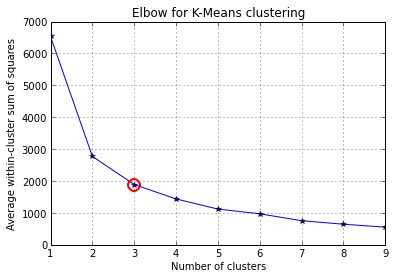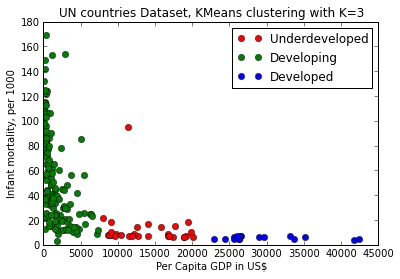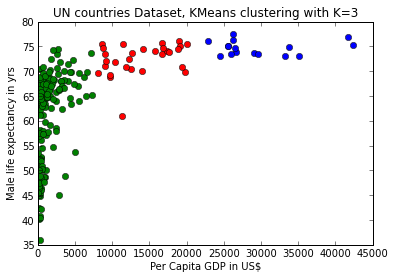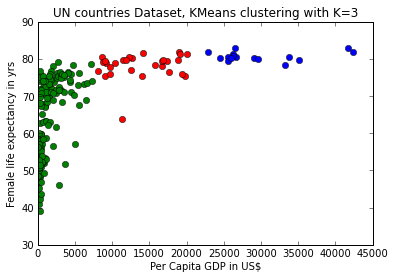## Results¶

In :
%pylab inline
# We run the following SciPy and NumPy code in 
# and generate the plots mentioned above using Matplotlib

# load the UN dataset transformed to float with 4 numeric columns,
# lifeMale,lifeFemale,infantMortality and GDPperCapita

fName = ('../datasets/UN4col.csv')
fp = open(fName)
fp.close()

Populating the interactive namespace from numpy and matplotlib

In :
import numpy as np
from scipy.cluster.vq import kmeans,vq
from scipy.spatial.distance import cdist
import matplotlib.pyplot as plt

##### cluster data into K=1..10 clusters #####
#K, KM, centroids,D_k,cIdx,dist,avgWithinSS = kmeans.run_kmeans(X,10)

K = range(1,10)

# scipy.cluster.vq.kmeans
KM = [kmeans(X,k) for k in K] # apply kmeans 1 to 10
centroids = [cent for (cent,var) in KM]   # cluster centroids

D_k = [cdist(X, cent, 'euclidean') for cent in centroids]

cIdx = [np.argmin(D,axis=1) for D in D_k]
dist = [np.min(D,axis=1) for D in D_k]
avgWithinSS = [sum(d)/X.shape for d in dist]

In :
kIdx = 2
# plot elbow curve
fig = plt.figure()
ax.plot(K, avgWithinSS, 'b*-')
ax.plot(K[kIdx], avgWithinSS[kIdx], marker='o', markersize=12,
markeredgewidth=2, markeredgecolor='r', markerfacecolor='None')
plt.grid(True)
plt.xlabel('Number of clusters')
plt.ylabel('Average within-cluster sum of squares')
tt = plt.title('Elbow for K-Means clustering')So we see that a good K to use in our model would be 3. We now use the KMeans modeling software to fit clusters to our data and then plot how the software has clustered our data. In this case we look at how the data cluster when we plot Infant Mortality against GDP.

In :
from sklearn.cluster import KMeans
km = KMeans(3, init='k-means++') # initialize
km.fit(X)
c = km.predict(X) # classify into three clusters

In :
# see the code in helper library kmeans.py
# it wraps a number of variables and maps integers to categoriy labels
# this wrapper makes it easy to interact with this code and try other variables
# as we see below in the next plot
import kmeans as mykm
(pl0,pl1,pl2) = mykm.plot_clusters(X,c,3,2) # column 3 GDP, vs column 2 infant mortality. Note indexing is 0 basedHere we see some patterns, obvious in retrospect. The countries with GDP (in US Dollars) below 10K have rapidly rising infant mortality as GDP drops. On the other hand as GDP rises we see rapidly decreasing infant mortality, which is as we know, a correlate of financial prosperity, i.e. high GDP.

We also see 3 clusters which we can informally call, the underdeveloped, the developing and the developed countries, based on, respectively, GDP (in US Dollars) below 10K, between 10K and 20K and finally greater than 20K.

What would happen if we tried other dimensions to cluster on, say lifeMale and GDPperCapita. Let's see.

In :
(pl0,pl1,pl2) = mykm.plot_clusters(X,c,3,0,False)And similarly with lifeFemale vs GDPperCapita.

In :
(pl0,pl1,pl2) = mykm.plot_clusters(X,c,3,1,False)In both the last two cases we see an opposite trend to infant mortality, where life expectancy rises rapidly as GDP grows, but drop precipitously even to below 40 yrs for countries with the lowest GDP.

Sections of code above are taken from a StackOverflow discussion .
Authorship of these segments is due to user Amro  on StackOverflow.
The discussion  has greater detail and more extensive examples and the reader is referred there for more depth.

### Exercise¶

• Look at the handwriting recognition dataset.
• Import it and run the code in the rest of the discussion.
• Do you get similar results?
In :
from IPython.core.display import HTML
def css_styling():